# Vector calculus

Vector calculus, or vector analysis, is concerned with differentiation and integration of vector fields, primarily in 3-dimensional Euclidean space The term "vector calculus" is sometimes used as a synonym for the broader subject of multivariable calculus, which spans vector calculus as well as partial differentiation and multiple integration. Vector calculus plays an important role in differential geometry and in the study of partial differential equations. It is used extensively in physics and engineering, especially in the description ofelectromagnetic fields, gravitational fields, and fluid flow. Vector calculus was developed from quaternion analysis by J. Willard Gibbs and Oliver Heaviside near the end of the 19th century, and most of the notation and terminology was established by Gibbs and Edwin Bidwell Wilson in their 1901 book, Vector Analysis. In the conventional form using cross products, vector calculus does not generalize to higher dimensions, while the alternative approach of geometric algebra which uses exterior products does (see below for more). (Wikipedia).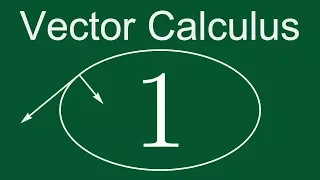Vector Calculus 1: What Is a Vector?

https://bit.ly/PavelPatreon https://lem.ma/LA - Linear Algebra on Lemma http://bit.ly/ITCYTNew - Dr. Grinfeld's Tensor Calculus textbook https://lem.ma/prep - Complete SAT Math Prep

From playlist Vector Calculus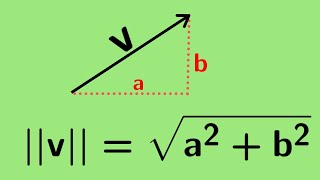Multivariable Calculus | The notion of a vector and its length.

We define the notion of a vector as it relates to multivariable calculus and define its length. http://www.michael-penn.net http://www.randolphcollege.edu/mathematics/

From playlist Vectors for Multivariable Calculus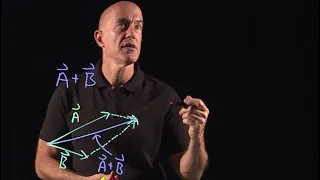Vectors | Lecture 1 | Vector Calculus for Engineers

Defines vectors, vector addition and vector subtraction. Join me on Coursera: https://www.coursera.org/learn/vector-calculus-engineers Lecture notes at http://www.math.ust.hk/~machas/vector-calculus-for-engineers.pdf Subscribe to my channel: http://www.youtube.com/user/jchasnov?sub_con

From playlist Vector Calculus for Engineers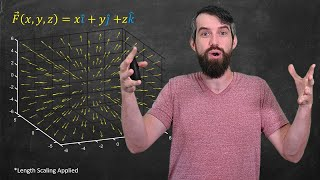Intro to VECTOR FIELDS // Sketching by hand & with computers

Vector Fields are extremely important in math, physics, engineering, and many other fields. Gravitational fields, electric fields, magnetic fields, velocity fields, these are all examples of vector fields. In this video we will define the concept of a vector field, talk about some basic teScalar and vector fields | Lecture 11 | Vector Calculus for Engineers

Definition of a scalar and vector field. How to visualize a two-dimensional vector field. Join me on Coursera: https://www.coursera.org/learn/vector-calculus-engineers Lecture notes at http://www.math.ust.hk/~machas/vector-calculus-for-engineers.pdf Subscribe to my channel: http://www

From playlist Vector Calculus for Engineers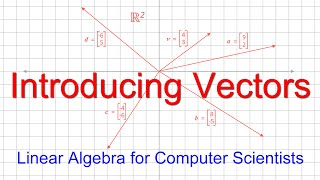Linear Algebra for Computer Scientists. 1. Introducing Vectors

This computer science video is one of a series on linear algebra for computer scientists. This video introduces the concept of a vector. A vector is essentially a list of numbers that can be represented with an array or a function. Vectors are used for data analysis in a wide range of f

From playlist Linear Algebra for Computer Scientists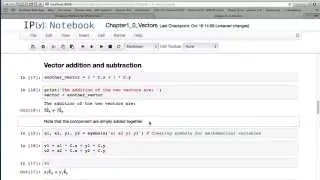1 Vectors

A short refresher on vectors. Before I introduce vector-based functions, it's important to look at vectors themselves and how they are represented in python™ and the IPython Notebook using SymPy.

From playlist Life Science Math: Vectors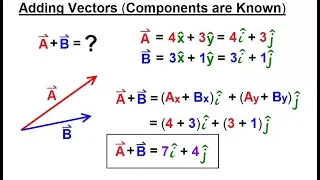Calculus 3: Vector Calculus in 2D (8 of 39) Adding Vectors (Components are Known)

Visit http://ilectureonline.com for more math and science lectures! In this video I will find the resultant vector given the components of vectors A and B are known. Next video in the series can be seen at: https://youtu.be/e1-OvKiLsho

From playlist CALCULUS 3 CH 3 VECTOR CALCULUS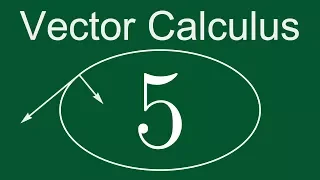Vector Calculus 5: Vector Equation of a Straight Line

https://bit.ly/PavelPatreon https://lem.ma/LA - Linear Algebra on Lemma http://bit.ly/ITCYTNew - Dr. Grinfeld's Tensor Calculus textbook https://lem.ma/prep - Complete SAT Math Prep

From playlist Vector Calculus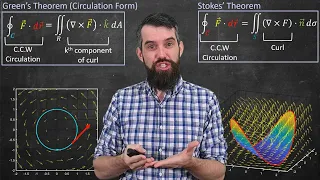A unified view of Vector Calculus (Stoke's Theorem, Divergence Theorem & Green's Theorem)

In the final video of my vector calculus playlist (congrats to everyone for making it to the end!!!) I want to do a bit of an overview of the major theorems we have seen in this course - Stokes' Theorem, Divergence Theorem, Green's Theorem - all are part of a unified framework. Loosely, in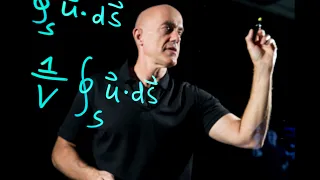Promotional Video | Vector Calculus for Engineers

My promotional video for my free-to-audit Coursera course, Vector Calculus for Engineers. Why should engineers learn vector calculus? Join me on Coursera: https://www.coursera.org/learn/vector-calculus-engineers Lecture notes at http://www.math.ust.hk/~machas/vector-calculus-for-engin

From playlist Vector Calculus for EngineersA random lecture from Calculus III

A random lecture from my Calculus III class from fall 2020. It follows Volume 4 of Calculus Illustrated by Peter Saveliev. See http://calculus123.com.

From playlist Calculus III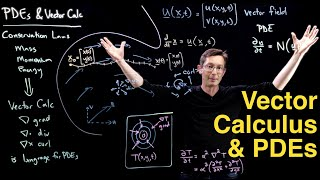Vector Calculus and Partial Differential Equations: Big Picture Overview

This video describes how vector calculus is the language we use to derive partial differential equations (PDEs) to encode physical laws, such as mass, momentum, and energy conservation in fluids. This is the first lecture of a series that will describe Div, Grad, and Curl; Gauss and StokeFind a Unit Vector in the Direction of a Given Vector with Length 4.

In this video we are given a vector and we are asked to find another vector in the same direction but with length 4. I hope this helps someone who is studying calculus and learning about vectors. Great Calculus Book: https://amzn.to/3VfotAU This is my affiliate link. As an Amazon Associa

From playlist Vectors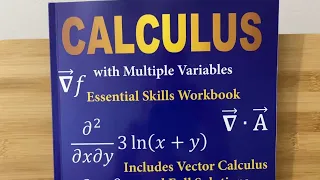The Ultimate Multivariable Calculus Workbook

In this video I will show you this amazing workbook which you can use to learn multivariable calculus. This workbook has tons of problems and includes full solutions to every single problem. Some of the topics covered include Partial Differentiation, The Chain Rule, Vector Calculus, Line I

From playlist Book Reviews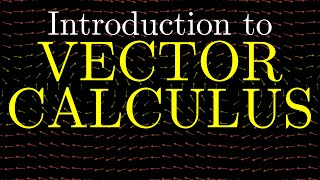INTRODUCTION to VECTOR CALCULUS calculus 3

This video is the first in a series of videos which serve as an introduction to vector calculus and calculus 3. These videos are intended as supplemental material for the course MATH2021 which I am presently teaching at the University of Sydney. 0:00 Introduction and chit chat 2:48 Main

From playlist All VideosFind a Unit Vector in the Direction of Another Vector

In this video we find a unit vector in the direction of another vector. I hope this helps someone who is studying calculus and learning about vectors. Great Calculus Book: https://amzn.to/3VfotAU This is my affiliate link. As an Amazon Associate I earn from qualifying purchases. If you en

From playlist Vectors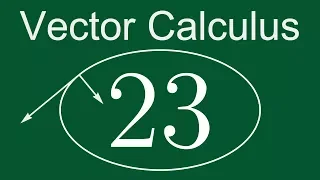Vector Calculus 23: The Laws of Vector Differentiation

https://bit.ly/PavelPatreon https://lem.ma/LA - Linear Algebra on Lemma http://bit.ly/ITCYTNew - Dr. Grinfeld's Tensor Calculus textbook https://lem.ma/prep - Complete SAT Math Prep

From playlist Vector Calculus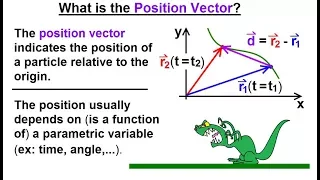Calculus 3: Vector Calculus in 2D (17 of 39) What is the Position Vector?

Visit http://ilectureonline.com for more math and science lectures! In this video I will explain what is the position vector. The position vector indicates the position of a particle relative to the origin. The position usually depends on, or is a function of, a parametric variable (ex. t

From playlist CALCULUS 3 CH 3 VECTOR CALCULUS